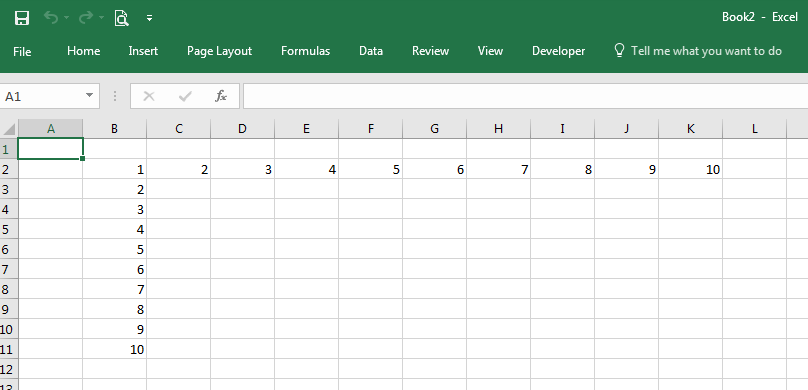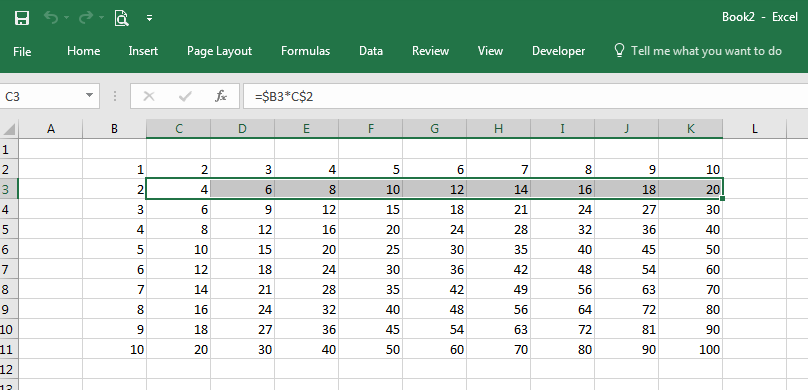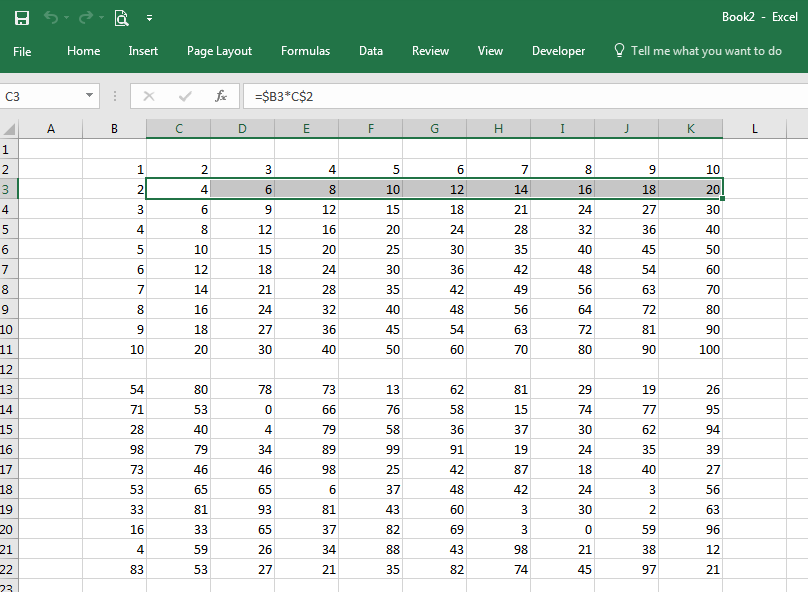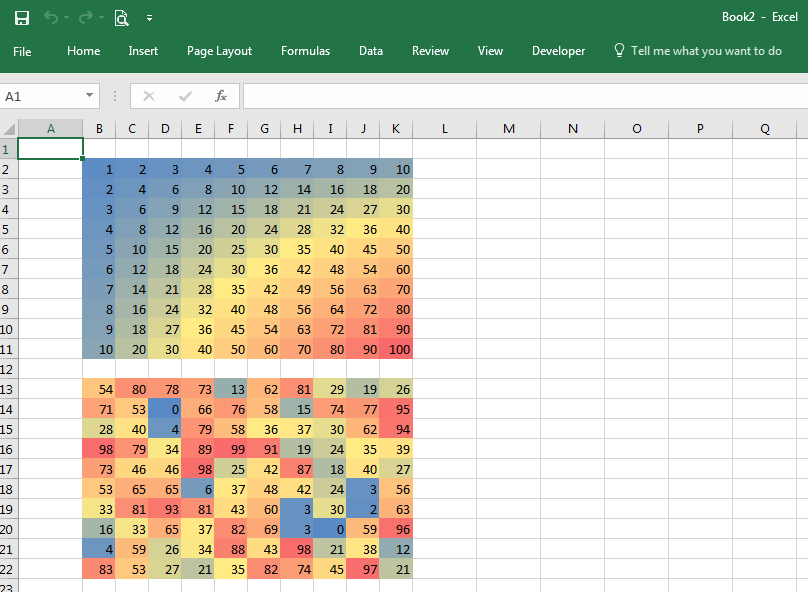The last post introduced a technique for recording a Visual Basic macro within Excel and porting it to Python. This exercise will build on those techniques while leveraging Python for more of the work. In this example, you will create two tables from scratch — a simple multiplication table and a table of random numbers — and apply conditional formatting to the numbers.

## Building the Complete Multiplication Table

To begin, start the IDLE tool and run the following commands to initialize the worksheet.

``````import win32com.client as win32
excel = win32.gencache.EnsureDispatch('Excel.Application')
excel.Visible = True
ws = wb.Worksheets('Sheet1')
``````

After typing these commands in IDLE, you’ll see an Excel window with an empty spreadsheet. To build the multiplication table, begin by populating the column and row headers. There are several ways to do this; for this exercise, you’ll pass a list of column header and a list of row header values to Excel. A row of data is defined by using the `ws.Range().Value` statement with a list or tuple on the right hand side of the equals sign. Rather than explicitly defining the list as `[1, 2, 3, 4, 5, 6, 7, 8, 9, 10]`, you can use Python’s list comprehension with a `range()` statement to populate the values as follows:

``````[i for i in range(1, 11)]
``````

The complete statement is ```ws.Range("B2:K2").Value = [i for i in range(1,11)]```. Defining a single column of data is a bit trickier; you must define a list of single-element lists or tuples. One way to do this is to use Python’s `zip()` function to transpose the flat list into a list of tuples. The complete statement is `ws.Range("B2:B11").Value = zip([i for i in range(1,11)])`. The statements for completing the column and row headers are shown below.

``````ws.Range("B2:K2").Value = [i for i in range(1, 11)]
ws.Range("B2:B11").Value = list(zip([i for i in range(1, 11)]))
``````To define the product values for each cell in the table, create a formula to multiply the column and row header for a single cell, then use Excel to autofill the remaining cells. Looking at the spreadsheet, the product for cell C3 is calculated by multiplying cell B3 by cell C2. For Excel, the formula is `=B3*C2`. To use Excel’s autofill capability in later steps, you need to anchor the row and column in the formula by prefixing it with the \$ character. In other words, the formula you want to use is `=\$B3*C\$2`. Once that formula is entered, the remaining cells can be filled in two steps. First, programmatically select the cell and drag it fill the row. Next, select the newly autofilled row and drag the new row down to fill in the remaining rows. Since this was demonstrated in the last post, please refer to that post if you need more information. The equivalent Python code to implement the autofill is shown below.

``````ws.Range("C3").Formula = "=\$B3*C\$2"
ws.Range("C3:C3").Select()
excel.Selection.AutoFill(ws.Range("C3:K3"),win32.constants.xlFillDefault)
ws.Range("C3:K3").Select()
excel.Selection.AutoFill(ws.Range("C3:K11"),win32.constants.xlFillDefault)
``````

The spreadsheet now contains the complete multiplication table.Now let’s add conditional formatting to the spreadsheet. Conditional formatting applies a color to individual cells based on the value of the cell, and helps you to spot outliers or discrepancies in a table of values.

Continuing with the same spreadsheet, we will create a second table of random integers between 1 and 100. Excel’s `RAND()` function will generate a random number between 0 and 1; to scale the values we’ll use the formula `=INT(RAND()*100)`. The `ws.Range().Formula` method can be used to fill a range with the same formula. The following code creates the table of random integers.

``````ws.Range("B13:K22").Formula = "=INT(RAND()*100)"
``````

The Excel spreadsheet should now contain both the multiplication and random number tables.Now you can apply conditional formatting to the multiplication table and the random table. Even though you invoked Excel from Python, you still can manipulate the spreadsheet using the Excel interface, and even record macros. Click “Record Macro” in the Developer tab to begin recording your macro to apply conditional formatting.

Select all the cells in the range B2:K22; both tables are included in the selection. In the Home tab, select Conditional Formatting->Color Scales->Red-Yellow-Blue Color Scale. If you can’t find the Red-Yellow-Blue Color Scale, choose another scale.

The spreadsheet should now show a color background for each of the selected cells with a value. Next, select columns B through K and set the column width to 4. Finally, select cell A1 and stop the macro by clicking “Stop Recording” in the Developer tab.

Your spreadsheet now has conditional formatting and will look something like this, with cells containing numbers near 100 colored in Red, cells with a value of 50 in Yellow, and cells with a value of 1 in Blue, with a shade of these colors for values in between:As an aside, you can update the random numbers in the lower table by hitting the F9 key to force Excel to reapply the formulas in the spreadsheet and calculate new random values.

To continue, open the macro just created by selecting Macros from the Developer tab, select the name of the macro you just captured and click Edit. The macro should look something like this:

``````Sub Macro1()
'
' Macro1 Macro
'
Range("B2:K22").Select
Selection.FormatConditions(Selection.FormatConditions.Count).SetFirstPriority
Selection.FormatConditions(1).ColorScaleCriteria(1).Type = _
xlConditionValueLowestValue
With Selection.FormatConditions(1).ColorScaleCriteria(1).FormatColor
.Color = 13011546
End With
Selection.FormatConditions(1).ColorScaleCriteria(2).Type = _
xlConditionValuePercentile
Selection.FormatConditions(1).ColorScaleCriteria(2).Value = 50
With Selection.FormatConditions(1).ColorScaleCriteria(2).FormatColor
.Color = 8711167
End With
Selection.FormatConditions(1).ColorScaleCriteria(3).Type = _
xlConditionValueHighestValue
With Selection.FormatConditions(1).ColorScaleCriteria(3).FormatColor
.Color = 7039480
End With
Range("A1").Select
End Sub
``````

Though the macro contains some very long method names and some `With` statements, the porting is straightforward. Here are some guidelines to keep in mind while migrating this code to Python:

• `Selection` is preceded by `excel.`

Remember that `Selection` is a method at the Excel Application level, you need to precede it with `excel`. in this example.

• Range is preceded by `ws`

`Range` is a method at the Worksheet level, which is defined earlier as `ws.` in this example.

• Function calls require `()` in Python

Unlike VB, any function calls must be followed by `()` in Python.

• `With` statements must be expanded

The three `With` blocks in this macro need to be expanded, which can be done with temporary variables or by copying the statement following the `With` keyword. For example, the first `With` block:

``````With Selection.FormatConditions(1).ColorScaleCriteria(1).FormatColor
.Color = 13011546
End With
``````

can be written in Python as

``````excel.Selection.FormatConditions(1).ColorScaleCriteria(1).FormatColor.Color = 13011546
``````

or by using a temporary variable as

``````x = excel.Selection.FormatConditions(1).ColorScaleCriteria(1).FormatColor
x.Color = 13011546
``````

or by using `with` as

``````with excel.Selection.FormatConditions(1).ColorScaleCriteria(1).FormatColor as fc:
fc.Color = 13011546
``````

Temporary variables were created to make the script more concise. In particular, the statement ```[csc1, csc2, csc3] = [excel.Selection.FormatConditions(1).ColorScaleCriteria(n) for n in range(1, 4)]``` was used to create three temporary variables for the three `ColorScaleCriteria` methods.

To save the spreadsheet and close Excel, use the `SaveAs` and `Quit` methods as shown in the full script example.

Here is the complete conditionalformatting.py script. The line `excel.Visible = True` has been commented out. Unless you are developing the script, you typically want Excel to run invisibly in the background.

conditionalformatting.py

``````#
# conditionalformatting.py
# Create two tables and apply conditional formatting
#
import win32com.client as win32
excel = win32.gencache.EnsureDispatch('Excel.Application')
# excel.Visible = True
ws = wb.Worksheets('Sheet1')
ws.Range("B2:K2").Value = [i for i in range(1, 11)]
ws.Range("B2:B11").Value = list(zip([i for i in range(1, 11)]))
ws.Range("C3").Formula = "=\$B3*C\$2"
ws.Range("C3:C3").Select()
excel.Selection.AutoFill(ws.Range("C3:K3"), win32.constants.xlFillDefault)
ws.Range("C3:K3").Select()
excel.Selection.AutoFill(ws.Range("C3:K11"), win32.constants.xlFillDefault)
# Add the table of random integers
ws.Range("B13:K22").Formula = "=INT(RAND()*100)"
ws.Range("B2:K22").Select()
excel.Selection.FormatConditions(excel.Selection.FormatConditions.Count).SetFirstPriority()
[csc1, csc2, csc3] = [excel.Selection.FormatConditions(1).ColorScaleCriteria(n) for n in range(1, 4)]
csc1.Type = win32.constants.xlConditionValueLowestValue
csc1.FormatColor.Color = 13011546
csc2.Type = win32.constants.xlConditionValuePercentile
csc2.Value = 50
csc2.FormatColor.Color = 8711167
csc3.Type = win32.constants.xlConditionValueHighestValue
csc3.FormatColor.Color = 7039480
ws.Range("B:K").ColumnWidth = 4
ws.Range("A1").Select()
wb.SaveAs('ConditionalFormatting.xlsx')
excel.Application.Quit()
``````

## Prerequisites

Python (refer to http://www.python.org)

pywin32 Python module https://pypi.org/project/pywin32

Microsoft Excel (refer to http://office.microsoft.com/excel)

## Source Files and Scripts

Source for the program conditionalformatting.py script is available at http://github.com/pythonexcels/examples

Originally Posted on October 20, 2009 / Updated November 1, 2022